GNSS Geodesy Innovation Interviews UAV/UAS Positioning Surveying Applications Perspective Time Navigation Mapping Policy Land Administration News Archives LBS GIS SDI Imaging E-Zine
 Geodesy

# Generalized orthogonalization an elegant solution to the least squares problem

Oct 2022 | No Comment

###### The generalized orthogonalization does not require the formation of the normal equations and is therefore numerically more stable than the conventional method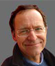## Abstract

The purpose of this article is to present a resolution method to the users of the least squares method in geodesy and topography and which has considerable advantages. This so-called “Generalized Orthogonalization” method is based on a modified version of the Gram-Schmidt orthogonalization method. It is very concise, numerically stable and allows the solution of simply or overdetermined systems of linear equations. It does not use any matrix inversion algorithm or the successive elimination technique. There is no formation of normal equations.

## Introduction

The least squares method was invented by Legendre to determine the orbital parameters of the planets. It was a little later that Gauss gave this method the foundations and rigorous mathematical formulations.

Since then, many mathematicians have contributed to the development and applications of this method. Let us quote Laplace, Tchebychev, Kalman and Markov. Significant work has also been carried out by geodesists such as Doolittle, Helmert, Tienstra, Meissl and Moritz. In Belgium we must refer to the works of Marchant, Baetslé and Van Den Herrewegen. We have also associated with certain algorithms, the name of those who proposed original methods allowing the numerical resolution associated with this method as in France Cholesky and Levallois. The other Banachiewicz, Gauss and Jordan are well known.

Today, the computer tools at our disposal require adapted numerical methods. It is agreed that they must meet a certain number of criteria including numerical stability, ease of programming, portability of the software, that is to say its independence to a specific operating system, etc.

The purpose of this paper is to present a method of resolution little known to users of the method of least squares in geodesy and surveying and which nevertheless has considerable advantages. This socalled “Generalized Orthogonalization” method is based on a modified version of the Gram-Schmidt orthogonalization method. It is very concise, numerically stable and allows the solution of simply or overdetermined systems of linear equations. It does not use any matrix inversion algorithm or the successive elimination technique. There is no formation of normal equations. The pioneers of this method are F. Charamza  and J. Gazdzicki .

We had already published an article on this method in 1986 in the Bulletin Trimestriel de la Société Belge de PhotogrammetrieTélédétection et Cartographie  when we worked at the National Geographic Institute of Belgium in the Department of Geodesy from 1983 to 1989. At the time the first mini-computers were appearing and the Department of Geodesy wanted to free itself from the services of the Computer Processing Center (CTI) which worked in time sharing on IBM servers at high prices charged per millisecond of CPU . To give an example, the processing of the aerial triangulation of Rwanda took 20 minutes and exhausted the annual budget of the CTI. This means that a certain independence was welcome.

Our mission was to develop a least squares adjustment software, including all the numerical refinements of the time, namely the B test method invented by Professor Baarda of Delft  for the detection of gross errors but also to produce the indicators internal and external reliability and the adjustment of free networks that call for generalized inversion.

Generalized orthogonalization was systematically used in our software at that time but also for all the software that we developed afterwards and in particular for example the Star Topo TX software from Star Informatic .

There was another request to allow the second-order design of a geodetic network where the position of the points is imposed and where one wishes, to achieve a given precision in terms of standard deviation, to calculate the precision to be obtained for each observation. Secondorder design is one of the most important orders in geodetic network design. In this design order, the optimal weights of the observations are sought. It also used a very particular matrix product invented by Khatri and Rao  used in a development by Gunther Schmitt .

At that time, there were very important international developments and contributions related to the numerical solution of the least squares problem. With limited means of digital processing, the imagination was highly solicited. Today the processing of millions of points from the famous point clouds generated by scanners and other sensors no longer surprises many people. On the other hand, students but also professionals in geodesy and surveying no longer seem motivated to dive into these algorithms with the risk of gradually losing their mastery and therefore becoming dependent on software production companies.

For my part, at that time, as a young graduate Surveyor-Expert with a background in Mathematics, I set myself the rule of professional life not to use any processing method that I could not both demonstrate and therefore program. This allowed me to understand exactly how the results of the observations were delivered and also to optimize the surveying operations and to innovate through the choice of mathematical models. In this respect, the arrival of GPS and then GNSS has been a godsend for many new developments, all of which are nevertheless based on this knowledge base of the least squares method coupled with digital filtering and statistical inference.

The teaching of mathematics, currently in a bad situation, aims, among other things, to discover a certain beauty. Bruno Hourst writes  Mathematics can be a source of beauty. We can speak of a “beautiful formula” or of a “beautiful geometric demonstration”. And indeed, when one has felt this beauty, it seems obvious. Geometric demonstrations are “beautiful” by the elegance of the reasoning and the articulation of the demonstration. Some formulas are “beautiful” by their simplicity or their balance.

Semir Zeki  explained: “The beauty of a formula can result from simplicity, symmetry, elegance or the expression of an immutable truth. For Plato, the abstract quality of mathematics expressed the pinnacle of beauty”.

Let’s quote Carl Friedrich Gauss again, about mathematics “The enchanting charms of this sublime science are revealed in all their beauty only to those who have the courage to explore it in depth. “

This article is therefore also an invitation to discover the elegance of an algorithm that takes another path to solve the problem of least squares. And indeed in the question of finding the minimum distance between a point and a line, one can either minimiser the distance function to this line or to construct the perpendicular of this point lowered on the line. Generalized orthogonalization is quite another way of solving the least squares problem by producing unbiased linear estimators in overdetermined systems of equations.

## Orthogonalization

Directions

We will present in this paragraph a reminder of the mathematical notions used in this article. Unless otherwise specified, all vectors will henceforth be column vectors.

When the components are explained, we will write [x1,x2,…,xn]T The symbol [ ]Tindicates that the elements must be written in columns.

n -component vectors over the set F is addition-stable, if the sum of any two of them is still a vector of the set. Similarly the set is stable for multiplication by the scalars if any vector of the set multiplied by a scalar is still a vector of the set.

Vector spaces

Any set of n – component vectors over F stable for multiplication by scalars and for addition is called a vector space .

Thus, if X1,X2,…,Xmare n-component vectors over F, the set of linear combinations

k1X1 + k2X2 … + kmXm with ki in F is a vector space over F.

The space Vn(F) of all n-component vectors over F is called the n-dimensional vector space over F.

Vn(R)will be the n-dimensional vector space over R, where R is the set of reals.

Subspaces

A set V of vectors of Vn(F) is a subspace of Vn(F) if V is stable for addition and multiplication by scalars. Thus, the n-dimensional non null vector space is a subspace of Vn(F). It is the same with Vn(F) himself.

Basis and Size

The dimension of a vector space V is the minimum number of linearly independent vectors to generate V. The set of these vectors is called a basis of V. If these are orthogonal two by two, the so-called orthogonal basis. If their norm is equal to unity, the basis will be said to be orthonormal.

In elementary geometry, the usual space is considered as a 3-dimensional set.

The so-called elementary vectors will be:

E1 =[1, 0, 0]T

E2=[0, 1, 0]T

E3 =[0, 0, 1]T

These constitute an important basis of V3(F)what is called the canonical basis of V3(F)

Gram-Schmidt orthogonalization

In linear algebra, in a pre-Hilbertian space (i.e. a vector space over the field of real numbers or that of complexes, endowed with a scalar product), the Gram-Schmidt process or algorithm is an algorithm for constructing , from a finite free family, an orthonormal basis of the subspace it generates. This method was published by Jørgen Pedersen Gram in 1883 and reformulated by Erhard Schmidt in 1907, but it is already found in works from 1816 by Laplace and by Cauchy in 1836 .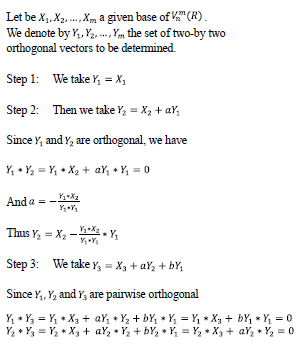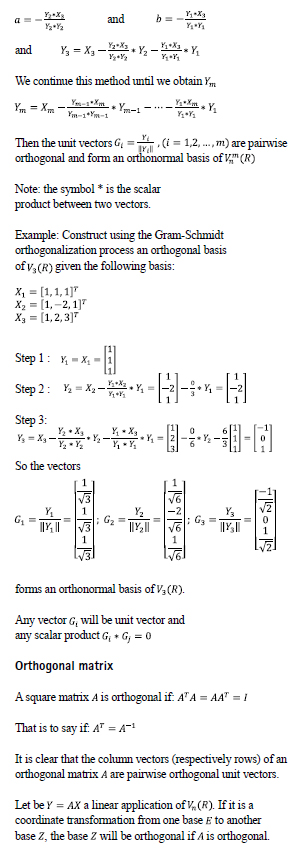The matrix formed by the vectors G1, G2 and G3of our previous example is orthogonal.

Orthogonalization and least squares method

Consider the problem of finding a vector belonging to Vn(R) such that AX = B where A belongs to Vnm(R) and B belongs to Vn(R)with n > m.

When there are more equations than unknowns, the system of equations is said to be overdetermined. The least squares problem consists in minimizing the Euclidean norm:

minx ||AX – B ||

A belonging to Vnm(R) and B belonging to Vn(R)

We ask for an optimal estimator for the vector X belonging to Vm(R).

The Euclidean norm being invariant with respect to orthogonal transformations, the least squares method benefits from the interesting property of being equivalent to the following problem:

minx||(QTA)X – (QTA)B)||

by multiplying A and B by an orthogonal matrix QTA).

Suppose that the orthogonal matrix QTA) has been calculated in such a way that: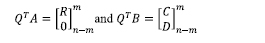The solution of the least squares problem is then obtained by solving the upper triangular system:

RX = C

Moreover the norm of the vector des residuals V = AX – B

will be equal to the norm of the vector .

In fact ||AX – B|2A) = ||QTAX – QTB||2 = ||RX – C||2 + D2

Three methods are generally proposed to calculate this orthogonal factorization. These are Gram-Schmidt orthogonalization, Householder reflectors and Givens rotations.

The method presented here is based on Gram-Schmidt orthogonalization. Unfortunately, this one a has a rather low numerical stability. To overcome this, an equivalent version called “modified Gram-Schmidt” has been proposed where the normalization of the vectors is done at each step of the transformation  and .

## Generalized orthogonalization

Directions

The generalized orthogonalization algorithm is based on the transformation of a matrix into a matrix of the same structure.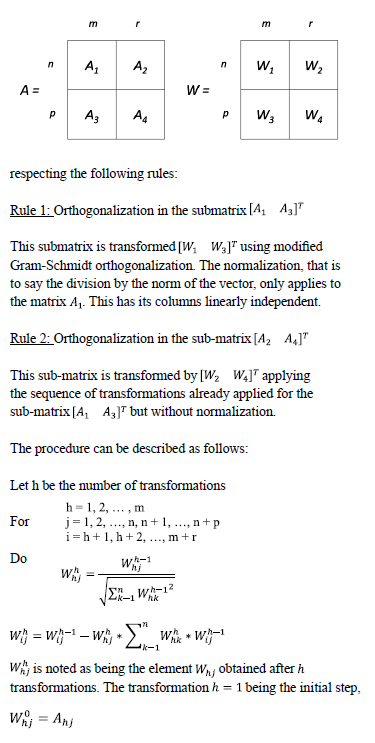Thus W1 becomes the orthonormal transformation of A1. We can verify that WT 1W1 = 1 . The sub-matrices A1 and W11 being a function of a non-singular right triangular matrix R such that

A1 = W1R

By applying the described procedure, we obtain the following fundamental relations of the generalized orthogonalization:

W1 = A1R-1 (1)

W3 = A3R-1 (2)

W2 = A2 – W1W1TA2 (3)

W4 = A4 – W3W1TA2 (4)

We will show later how to define the matrices A1, A2, A3and to obtain in a variety of cases the solution to the problems posed by the resolution of a simply determined or overdetermined system of linear equations.

System of simply determined linear equations

Either to determine the vector X in the equation AX = B

The solution is obtained by multiplying both sides of the equation by the inverse matrix A-1. Either A-1AX = A-1B and therefore X = A-1B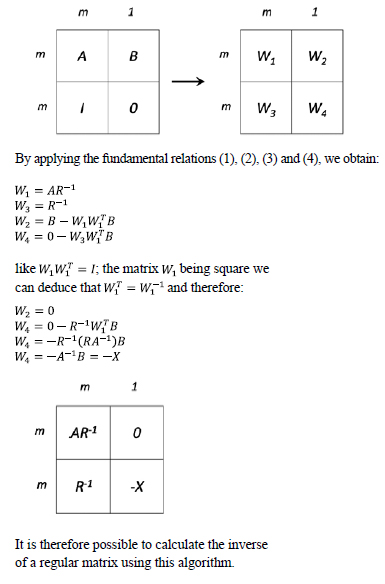It is therefore possible to calculate the inverse of a regular matrix using this algorithm.

System of overdetermined linear equations

Depending on whether the observations are expressed as a function of certain parameters (coordinates) or whether certain conditions are imposed on them, we will have different models of equations, leading however to identical results.

These are the model of observation equations (Gauss-Markov) and the model of condition equations (Gauss-Helmert).

One can be reduced to the other by algebraic elimination of relations between unmeasured quantities, but this is a problem of only theoretical interest  and .

There are obviously many other models adapted to more complex situations but which are only a category relating to one or other of the models mentioned.

Note also that due to the nature of the observations that are processed in topography and geodesy, certain established functions are not linear (directions/angles and distances). The implementation of the method of least squares requires that these equations be made linear by approximation by replacing them with a series expansion limited to linear terms.

Observation equations

We will briefly remind the general equations:

Consider the following linear functional model:

L = V = AX = A0 (5)

Where L is the vector of observations (n)

V is the vector of residuals (n)

A is the matrix of the coefficients of the observation equations (nxm)

X is the vector of the parameters to be estimated (m)

A0 is the vector of constant terms (n) integrated in practice into the vector L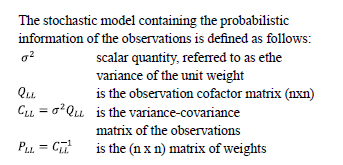scalar quantity, referred to as ethe variance of the unit weight is the observation cofactor matrix (nxn) is the variance-covariance matrix of the observations is the (n x n) matrix of weight

We obtain the following estimators:

For parameters: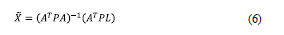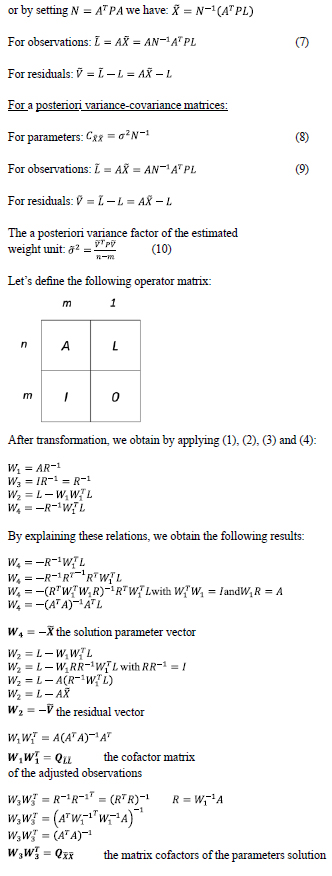We thus find after transformation in the matrix operator, the following information: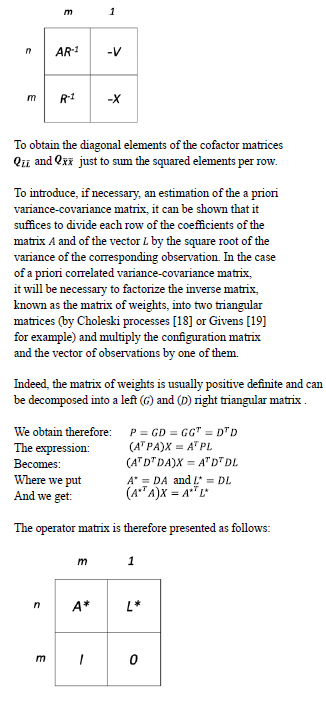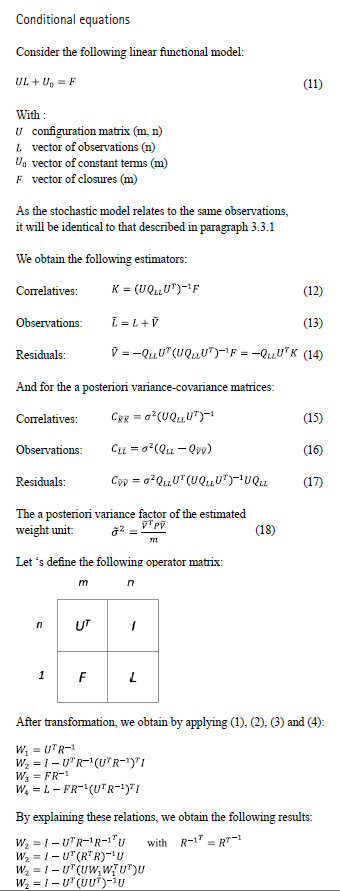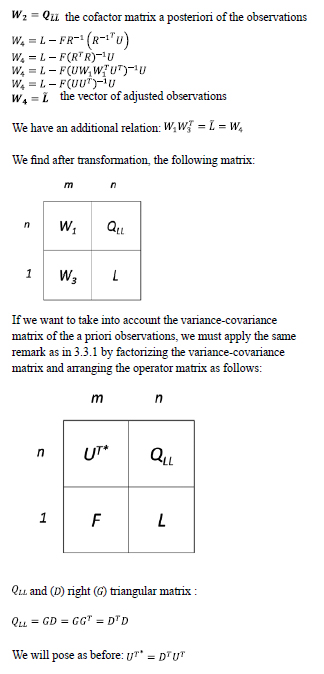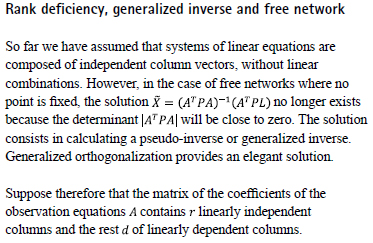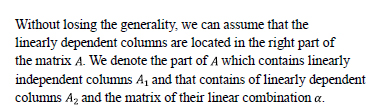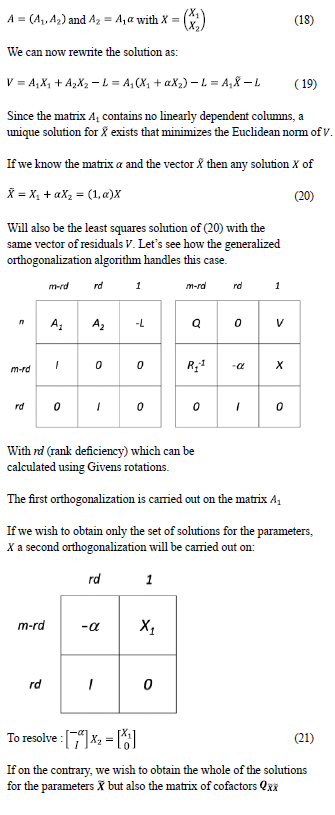.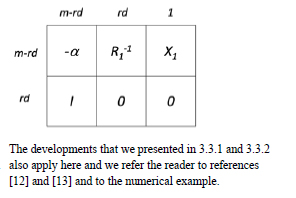## Numerical example

We propose in this paragraph a numerical example, both to illustrate our presentation and to offer readers wishing to test their own software a numerical basis of comparison.

Leveling Network

To illustrate the duality between the two models (GaussMarkov and Gauss Helmert), we will handle that example by the observation equations and the condition equations. Consider the leveling network represented by the following diagram: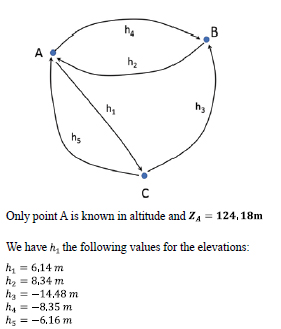The distance between points A, B and C will be equal to unity to simplify the stochastic model and we only deal with 2 decimals for the observations.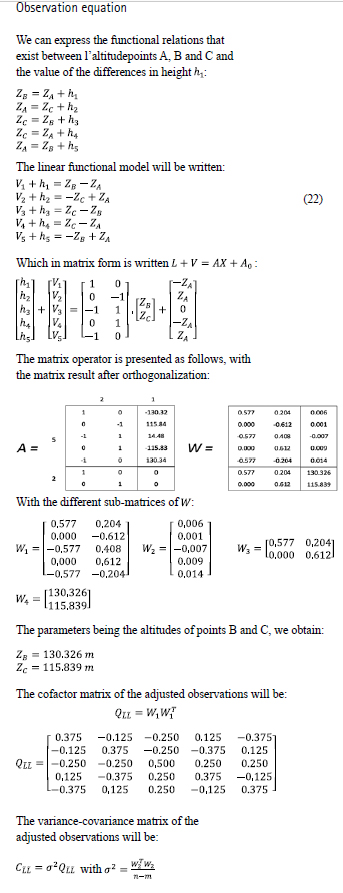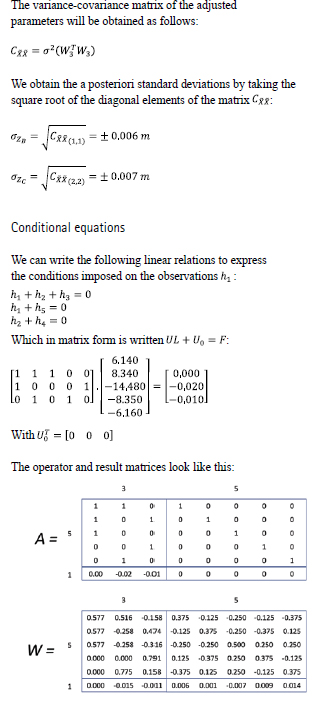One notes the perfect similarity between the model of the observation equations and the model of the conditioned equations with regard to the numerical results obtained for L and QLL

Rank deficiency, generalized inverse and free network

In the example of the leveling network, let’s ignore the dimension of point A. This is a free network adjustment (without constraint) whose interest is to focus on the observations. One could say that the model with condition equations also fulfill this role except that the automation of the closures is not easy. As such, the model with observation equations is easier to program since for each observation we have an equation.

In our example leveling network, we only have one known point in elevation and we can refer to this adjustment as a minimum constraint adjustment.

The functional model is written as follows, considering the altitude of point A as a parameter to be estimated: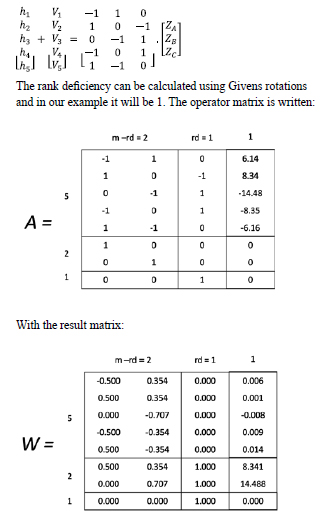It can be seen that the vector of residuals is identical to that obtained by the other models (observation equations and conditioned equations).

Moreover, and as mentioned before, the singular column relates to the last parameter which is the dimension of Point C. We can indeed re-arrange the parameters and make this singularity relate to the parameter of our choice.

We will proceed to the second orthogonalization which will relate to the lower part of the matrix operator is: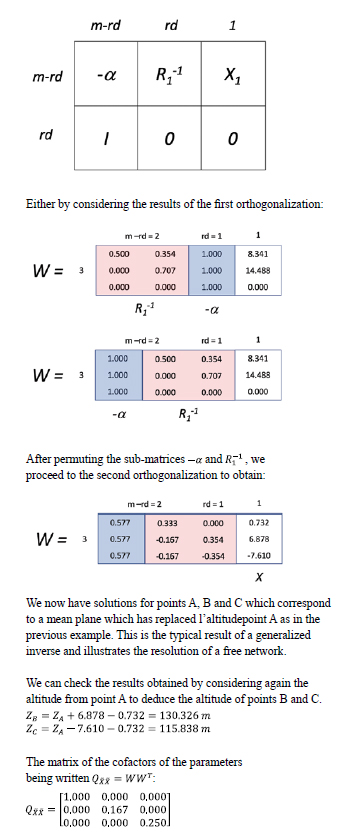This result further illustrates one of the attractions of free network adjustment since the parameter cofactor matrix is devoid of correlation.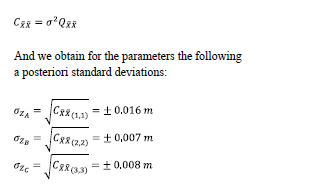Free network adjustment allows to conduct error detection tests, such as those we use and which are based on Professor Baarda’s B method , and also to test the congruence of control points.

This is the typical approach used for deformation networks in digital monitoring where the stability of the control points must also be tested before performing a constraint adjustment.

## Algorithm

The programming of the generalized orthogonalization algorithm does not offer any particular difficulty and we give an example written in ANSI C language.

As input, we need a matrix whose dimensions are as follows: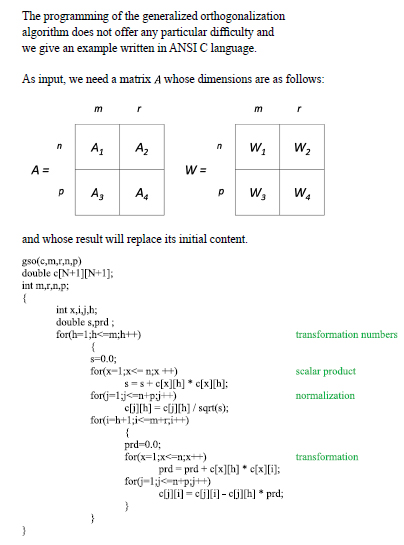## Conclusion

At the end of this article, we hope that the reader has been able to take into consideration the beauty and elegance of this algorithm. The conciseness of this one and the fact that the transformations are carried out in the initial matrix operator, allows us to use it abundantly in all the software applications that we develop where we have to apply the method of least squares adjustment and the method B test invented by Professor Baarda of Delft .

The generalized orthogonalization does not require the formation of the normal equations and is therefore numerically more stable than the conventional method . In addition, it can handle all equation models as well as the adjustment of free networks that are common in monitoring and deformation study projects.

We would like to thank Aleš Čepek from the Czech Technical University in Prague for our recent discussions and exchanges on generalized orthogonalization. He was the collaborator of F. Charamza and one of the authors of the free software GNU GAMA which is dedicated to the adjustment of topographic networks. This software also uses generalized orthogonalization.

## References

 F. Charamza, Orthogonalization Algorithm for solving the fundamental problems of the calculus of observations. Geofysikali Sbornik XIX 1971 No. 346. Academy Praha.

 J. Gazdzicki, Nowe algorytmy metoda najmniejszych kwadratow (New Algorithms of Adjustment by the Method of Least Squares), in Prace instytutu Geodezji i Kartografii, Tom XIII Zestyt 2(29) Warsawa 1966.

 Joël van Cranenbroeck, 1986, Generalized Orthogonalization, An Algorithmic Solution to the Least Squares Problem, Quarterly Bulletin of the Belgian Society for PhotogrammetryRemote Sensing and Cartography N°163- 164, September-December 1986

 CG Khatri and C. Radhakrisna Rao, Solutions to some Functional Equations and their applications to characterization of Probability Distributions in Sankhya : The Indian Journal of Statistics 1968, Volume 30, Series A, Pt. 2, pp. 167—180

 Schmitt, G., 1985. Second order design, In: Grafarend, E.W., Sanso, F. (editors) Optimization and design of geodetic networks. Springer, Berlin, Heidelberg, New York.

 Bruno Hourst sur https://www.mieux-apprendre.com/ blog/2018/04/02/mathematiques-art-et-beaute/

 https://en.wikipedia.org/wiki/Gram– Schmidt_process?oldid=14454636

 A. Björk, Solving Linear Least Squares problems by Gram-Schmidt orthogonalization. BIT 7 (1967), 1-21

 G.H. Golub & C.F. Van Loan, Matrix Computations. (1983) John Hopkins University Press, Baltimore

 J. Adam, A detailed Study of the duality Relation for Least-Squares Adjustment in Euclidean Spaces. Bulletin Géodésique, Vol. 56, n°3, pp 180-195.

 J.A.R. Blais, Duality Considerations in Linear Least Squares. Manuscripta Geodaetica, Vol. 8, (1983), pp 199-213.

 F. Charamza, 1978. An Algorithm for the Minimum-Length Least Squares Solution of a Set of Observation Equations, Presented at the I.A.G. International Symposium of Optimization of Design and Computation of Control Network, Sopron, Hungary 1977.

 Aleš Čepek, J. Pytel, 2005. A Progress Report on Numerical Solutions of Least Squares Adjustment in GNU Project Gama. Acta Polytechnica Vol. 45 N01/2005 by Czech Technical University in Prague.

 T. Krarup – The Theory of Rounding Errors in the Adjustment by Elements of Geodetic Networks in 2nd International Symposium on Geodetic Calculations – June 1966

 W. Baarda – A Testing Procedure for Use in Geodetic Networks 1968

 https://www.rtbf.be/article/le-groupe-anglais-1spatialrachete-la-societe-liegeoise-star-apic-8020816

 http://images.math.cnrs.fr/Du-cote-deslettres-une-lettre-de-Sophie-Germaina-Carl-Friedrich-Gauss-20.html

 https://fr.wikipedia.org/wiki/Factorisation_de_Cholesky

 https://en.wikipedia.org/wiki/Givens_rotation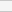(No Ratings Yet)Loading...